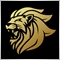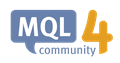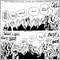# How to resize 2d array?125

Hello. I'm trying to resize 2d array.
```double array;
ArrayResize(array, 100, 100);
Print(array);```

Could anybody help me?

I'm trying it in MQL4Moderator
12773

https://docs.mql4.com/array/arrayresize

The function can only be applied to dynamic array's.ArrayResize - Array Functions - MQL4 Reference
• docs.mql4.com
The function can be applied only to dynamic arrays. It should be noted that you cannot change the size of dynamic arrays assigned as indicator buffers by the SetIndexBuffer() function. For indicator buffers, all operations of resizing are performed by the runtime subsystem of the terminal. With the frequent memory allocation, it is recommended...125

Marco vd Heijden:

https://docs.mql4.com/array/arrayresize

The function can only be applied to dynamic array's.

https://docs.mql4.com/basis/types/dynamic_array

Here is written: "Maximum 4-dimension array can be declared." and also example:

```double matrix[]; // 3-dimensional dynamic array
ArrayResize(matrix,5);   // Set the size of the first dimension
```

so i need to change not 1st dimension's size but 2nd's.Dynamic Array Object - Data Types - Language Basics - MQL4 Reference
• docs.mql4.com
Maximum 4-dimension array can be declared. When declaring a dynamic array (an array of unspecified value in the first pair of square brackets), the compiler automatically creates a variable of the above structure (a dynamic array object) and provides a code for the correct initialization. Static Arrays When all significant array dimensions are...Moderator
12773

```double array;
ArrayResize(array,```

Shows a static array so not a dynamic.715

Revazi Tchitanava:

https://docs.mql4.com/basis/types/dynamic_array

Here is written: "Maximum 4-dimension array can be declared." and also example:

so i need to change not 1st dimension's size but 2nd's.

You can't resize the 2nd dimension of a multi dimensional array.

Only the first dimension can be changed.

Its a better to convert your 2-dimension array (maxtrix)

into a linear 1 dimensional array.

--------------------------------------------------------------

1e dimension = y | array index nr. for number of rows, start with 0

2e dimension = x | array index nr. for number of columns, start with 0

max width = max number of elements in the second dimension (x) your example ArrayResize(array, 100, 100) = max 100 elements

index number formula = (y * WIDTH) + x

You can now use the ArrayResize() function to change the size of the array

In your example ArrayResize(matrix, 100 * 100);

Greetings125

Snelle Moda:

You can't resize the 2nd dimension of a multi dimensional array.

Only the first dimension can be changed.

Its a better to convert your 2-dimension array (maxtrix)

into a linear 1 dimensional array.

--------------------------------------------------------------

1e dimension = y | array index nr. for number of rows, start with 0

2e dimension = x | array index nr. for number of columns, start with 0

max width = max number of elements in the second dimension (x) your example ArrayResize(array, 100, 100) = max 100 elements

index number formula = (y * WIDTH) + x

You can now use the ArrayResize() function to change the size of the array

In your example ArrayResize(matrix, 100 * 100);

Greetings

could you gave code example? compilable one. please. thank you.715

Revazi Tchitanava:

could you gave code example? compilable one. please. thank you.

this example copies 10 CLOSE prices of a 1 minute and 1 day BARS to a data array and prints the values on the screen.

```   int numberOfBars       = 10; // y axis | rows    | history BARS
int numberOfPeriods    = 2;  // x axis | columns | PERIOD_M1 and PERIOD_D1

ENUM_TIMEFRAMES period1_M1 = PERIOD_M1; // minute Bars
ENUM_TIMEFRAMES period2_D1 = PERIOD_D1; // day bars

double total_Data_Array[]; // array for both data sets
ArrayResize(total_Data_Array, numberOfBars * numberOfPeriods);

double a1[]; // temp array for data set 1
CopyClose(Symbol(), period1_M1, 1, numberOfBars, a1);

double a2[]; // temp array for data set 2
CopyClose(Symbol(), period2_D1, 1, numberOfBars, a2);

for(int y = 0; y < numberOfBars; y++)
{
// combine both array's
int index1 = (y * numberOfPeriods) + 0; // data m1 period
total_Data_Array[index1] = a1[y];

int index2 = (y * numberOfPeriods) + 1; // data D1 period
total_Data_Array[index2] = a2[y];
}

for(int y = 0; y < numberOfBars; y++)
{
for(int x = 0; x < numberOfPeriods; x++)
{
int arrayIndexNr = (y * numberOfPeriods) + x;
Alert("Close price for period: ", (x + 1), " = ", total_Data_Array[arrayIndexNr]);
}
}
```